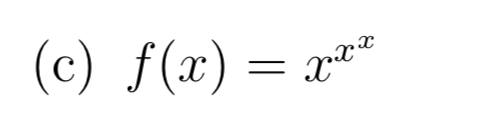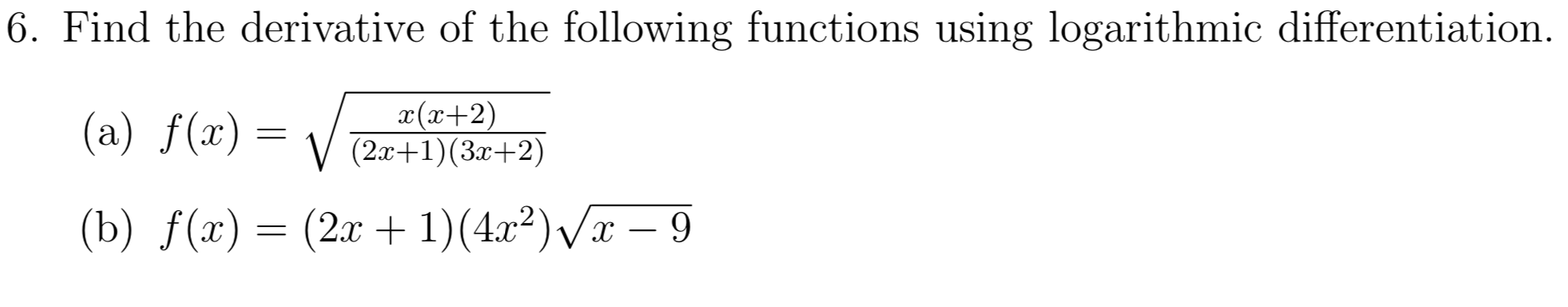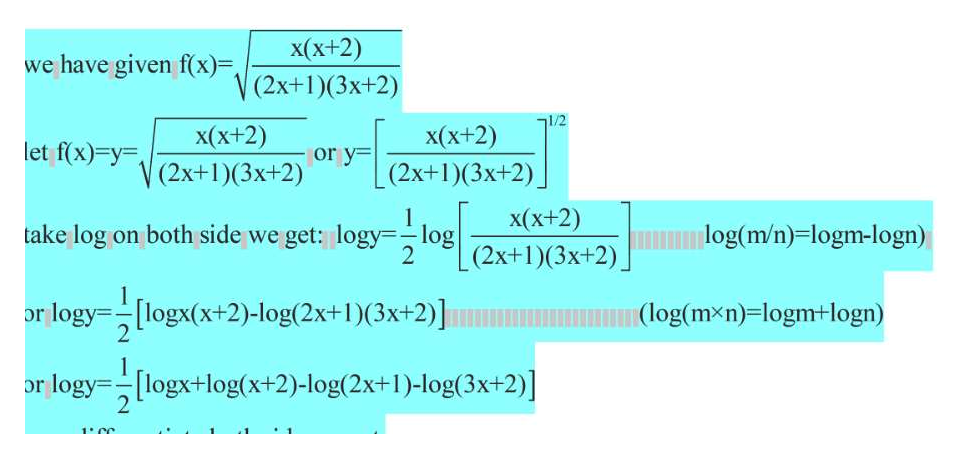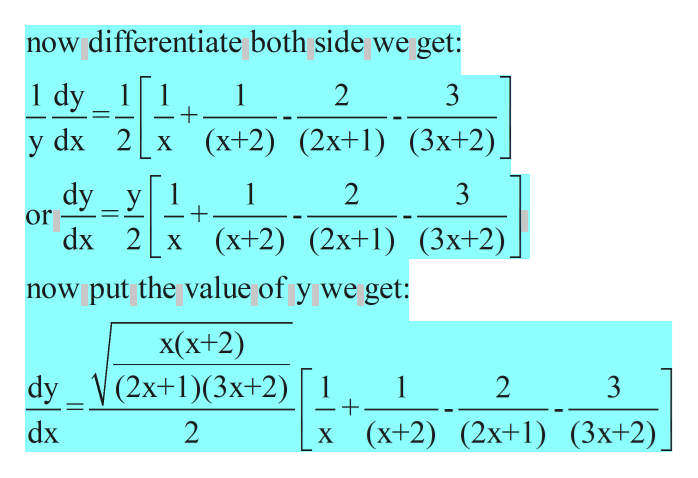# (c) f(x) = x |6. Find the derivative of the following functions using logarithmic differentiation.x(x+2)(2x+1) (3+2)(a) f(x)(2r1)(422)x - 9(b) f(x)

Question
1 viewshelp_outlineImage Transcriptionclose(c) f(x) = x fullscreenhelp_outlineImage Transcriptionclose|6. Find the derivative of the following functions using logarithmic differentiation. x(x+2) (2x+1) (3+2) (a) f(x) (2r1)(422)x - 9 (b) f(x) fullscreen
check_circle

Step 1

take log on both side and using the property.help_outlineImage Transcriptionclosex(x+2) we have given f(x), V(2x+1)(3x+2) 71/2 x(x+2) x(x+2) let f(x) y or y (2x+1)(3x+2) (2x+1)(3x+2) x(x+2) (2x+1)(3x+2) take log on both side we get: logy-log 2 log(m/n)=logm-logn) or logylogx(x+2)-log(2x+1)(3x+2)] (log(mxn)-logm+logn) 1 or logylogx+log(x+2)-log(2x+1)-log(3x+2)] fullscreen
Step 2

differentiation of logarithmic function.help_outlineImage Transcriptionclosenow differentiate both side we get: 1 dy 1 2 3 dx 2 x (x+2) (2x+1) (3x+2) У dy_y 1 dx 2x (х+2) (2x+1) (3х+2) 2 1 3 or now put the value of y we get: x(х+2) dy_V(2x+1)(3x+2) 1 1 + 2 3 х (x+2) (2х+1) (3x+2). dx 2 fullscreen
Step 3

take log on both side and u...

### Want to see the full answer?

See Solution

#### Want to see this answer and more?

Solutions are written by subject experts who are available 24/7. Questions are typically answered within 1 hour.*

See Solution
*Response times may vary by subject and question.
Tagged in

### Calculus